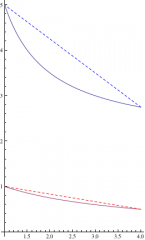Find the average rate of change for the function over the given interval. Y = 3/x+2 between x = 1 and x = 4?∆y/∆x = ((3/4+2) - (3/1+2))/(4 - 1)
= (2 3/4 - 5)/3
= (-2 1/4)/3
= (-9/4)/3 = -3/4
_____
If you mean
y = 3/(x+2)
you will get a different result.
∆y/∆x = ((3/(4+2)) - (3/(1+2))/(4 - 1)
= (3/6 - 3/3)/3
= (1/2 - 1)/3
= (-1/2)/3
= -1/6
thanked the writer.